# Random Geometry Generation with PostGIS

A user on the postgis-users had an interesting question today: how to generate a geometry column in PostGIS with random points, linestrings, or polygons?

Random data is important for validating processing chains, analyses and reports. The best way to test a process is to feed it inputs!

## Random Points

Random points is pretty easy -- define an area of interest and then use the PostgreSQL random() function to create the X and Y values in that area.

``````CREATE TABLE random_points AS
WITH bounds AS (
SELECT 0 AS origin_x,
0 AS origin_y,
80 AS width,
80 AS height
)
SELECT ST_Point(width  * (random() - 0.5) + origin_x,
height * (random() - 0.5) + origin_y,
4326)::Geometry(Point, 4326) AS geom,
id
FROM bounds,
generate_series(0, 100) AS id
``````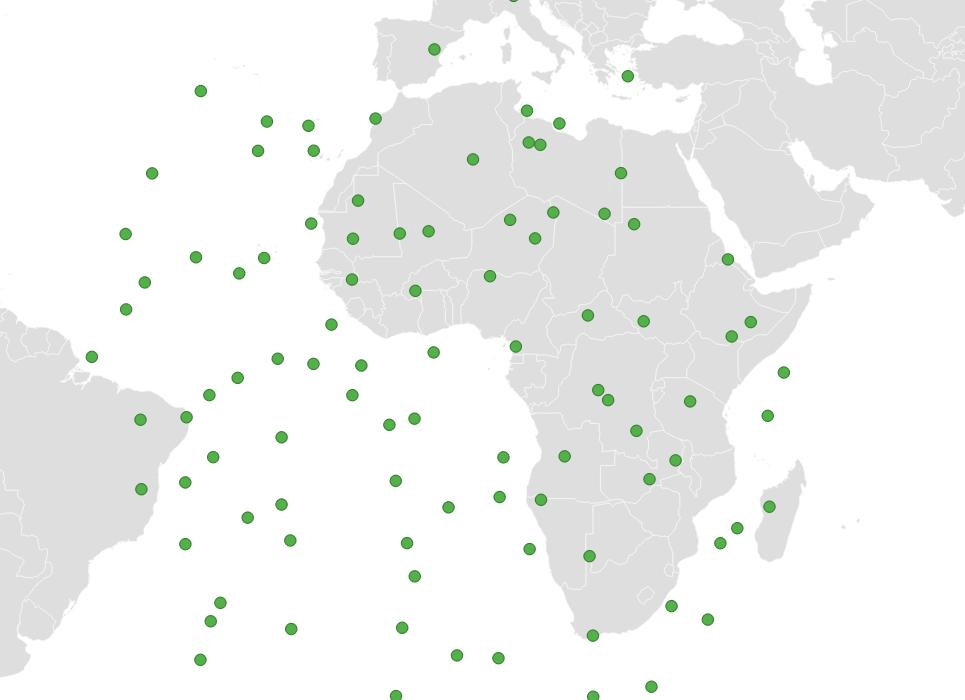Filling a target shape with random points is a common use case, and there's a special function just for that, ST_GeneratePoints(). Here we generate points inside a circle created with ST_Buffer().

``````CREATE TABLE random_points AS
SELECT ST_GeneratePoints(
ST_Buffer(
ST_Point(0, 0, 4326),
50),
100) AS geom
``````

If you have PostgreSQL 16, you can use the brand new random_normal() function to generate coordinates with a central tendency.

``````CREATE TABLE random_normal_points AS
WITH bounds AS (
SELECT 0 AS origin_x,
0 AS origin_y,
80 AS width,
80 AS height
)
SELECT ST_Point(random_normal(origin_x, width/4),
random_normal(origin_y, height/4),
4326)::Geometry(Point, 4326) AS geom,
id
FROM bounds,
generate_series(0, 100) AS id
``````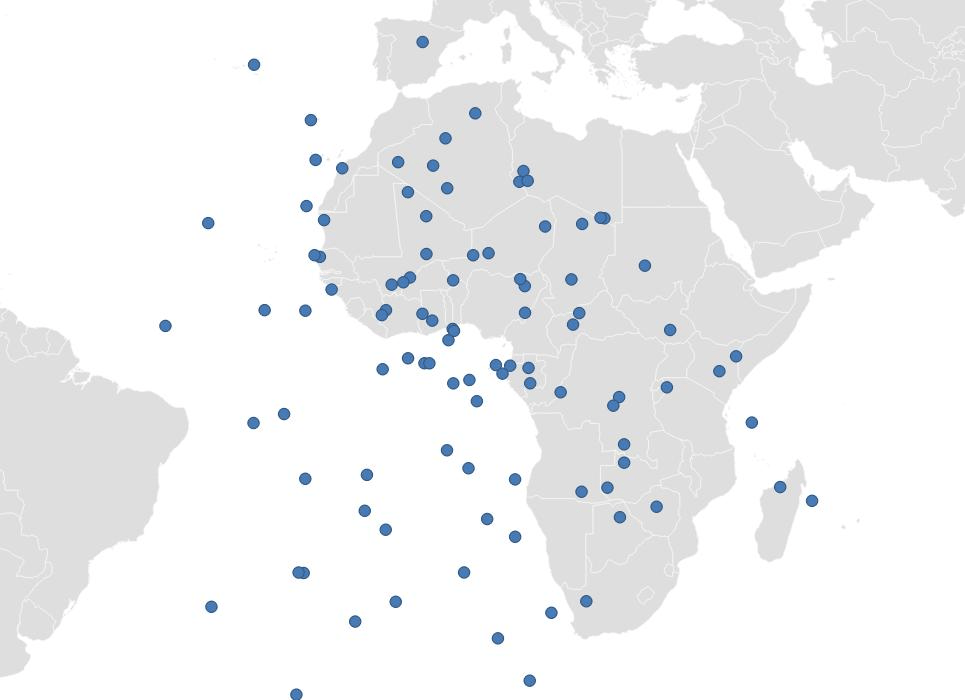For PostgreSQL versions before 16, here is a user-defined version of random_normal().
``````CREATE OR REPLACE FUNCTION random_normal(
mean double precision DEFAULT 0.0,
stddev double precision DEFAULT 1.0)
RETURNS double precision AS
\$\$
DECLARE
u1 double precision;
u2 double precision;
z0 double precision;
z1 double precision;
BEGIN
u1 := random();
u2 := random();

z0 := sqrt(-2.0 * ln(u1)) * cos(2.0 * pi() * u2);
z1 := sqrt(-2.0 * ln(u1)) * sin(2.0 * pi() * u2);

RETURN mean + (stddev * z0);
END;
\$\$ LANGUAGE plpgsql;
``````

## Random Linestrings

Linestrings are a little harder, because they involve more points, and aesthetically we like to avoid self-crossings of lines.

Two-point linestrings are pretty easy to generate with ST_MakeLine() -- just generate twice as many random points, and use them as the start and end points of the linestrings.

``````CREATE TABLE random_2point_lines AS
WITH bounds AS (
SELECT 0 AS origin_x, 80 AS width,
0 AS origin_y, 80 AS height
)
SELECT ST_MakeLine(
ST_Point(random_normal(origin_x, width/4),
random_normal(origin_y, height/4),
4326),
ST_Point(random_normal(origin_x, width/4),
random_normal(origin_y, height/4),
4326))::Geometry(LineString, 4326) AS geom,
id
FROM bounds,
generate_series(0, 100) AS id
``````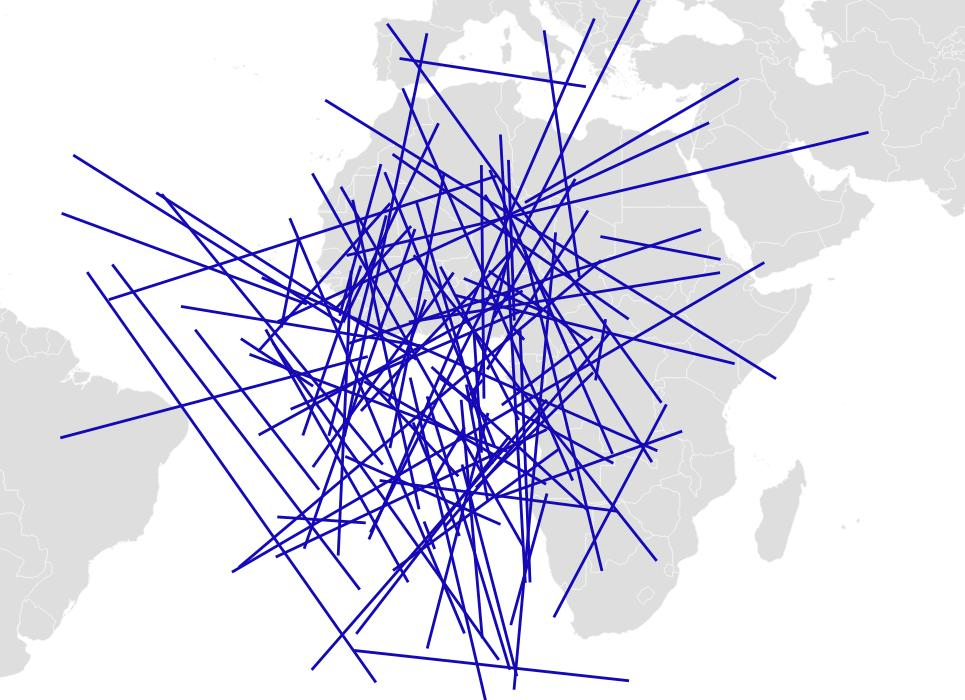Multi-point random linestrings are harder, at least while avoiding self-intersections, and there are a lot of potential approaches. While a recursive CTE could probably do it, an imperative approach using PL/PgSQL is more readable.

The `generate_random_linestring()` function starts with an empty linestring, and then adds on new segments one at a time, changing the direction of the line with each new segment.

Here is the full generate_random_linestring() definition.
``````CREATE OR REPLACE FUNCTION generate_random_linestring(
start_point geometry(Point))
RETURNS geometry(LineString, 4326) AS
\$\$
DECLARE
num_segments integer := 10; -- Number of segments in the linestring
deviation_max float := radians(45); -- Maximum deviation
random_point geometry(Point);
deviation float;
direction float := 2 * pi() * random();
segment_length float := 5; -- Length of each segment (adjust as needed)
i integer;
result geometry(LineString) := 'SRID=4326;LINESTRING EMPTY';
BEGIN
FOR i IN 1..num_segments LOOP
-- Generate a random angle within the specified deviation
deviation := 2 * deviation_max * random() - deviation_max;
direction := direction + deviation;

-- Calculate the coordinates of the next point
random_point := ST_Point(
ST_X(start_point) + cos(direction) * segment_length,
ST_Y(start_point) + sin(direction) * segment_length,
ST_SRID(start_point)
);

-- Add the point to the linestring

-- Update the start point for the next segment
start_point := random_point;

END LOOP;

RETURN result;
END;
\$\$
LANGUAGE plpgsql;
``````

We can use the `generate_random_linestring()` function now to turn random start points (created in the usual way) into fully random squiggly lines!

``````CREATE TABLE random_lines AS
WITH bounds AS (
SELECT 0 AS origin_x, 80 AS width,
0 AS origin_y, 80 AS height
)
SELECT id,
generate_random_linestring(
ST_Point(random_normal(origin_x, width/4),
random_normal(origin_y, height/4),
4326))::Geometry(LineString, 4326) AS geom
FROM bounds,
generate_series(1, 100) AS id;
``````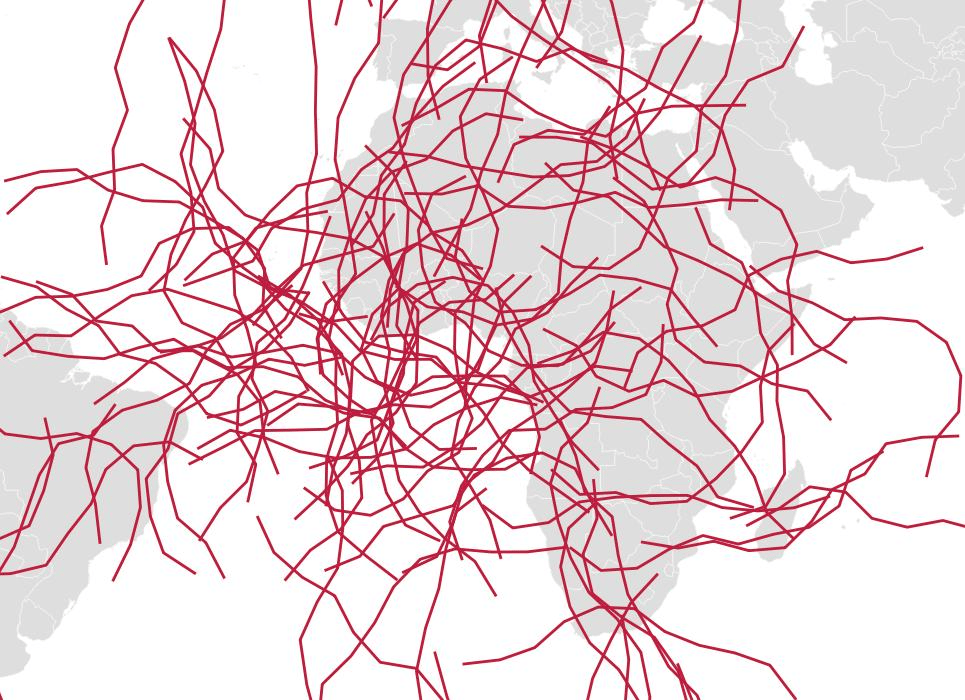## Random Polygons

At the simplest level, a set of random boxes is a set of random polygons, but that's pretty boring, and easy to generate using ST_MakeEnvelope().

``````CREATE TABLE random_boxes AS
WITH bounds AS (
SELECT 0 AS origin_x, 80 AS width,
0 AS origin_y, 80 AS height
)
SELECT ST_MakeEnvelope(
random_normal(origin_x, width/4),
random_normal(origin_y, height/4),
random_normal(origin_x, width/4),
random_normal(origin_y, height/4)
)::Geometry(Polygon, 4326) AS geom,
id
FROM bounds,
generate_series(0, 20) AS id

``````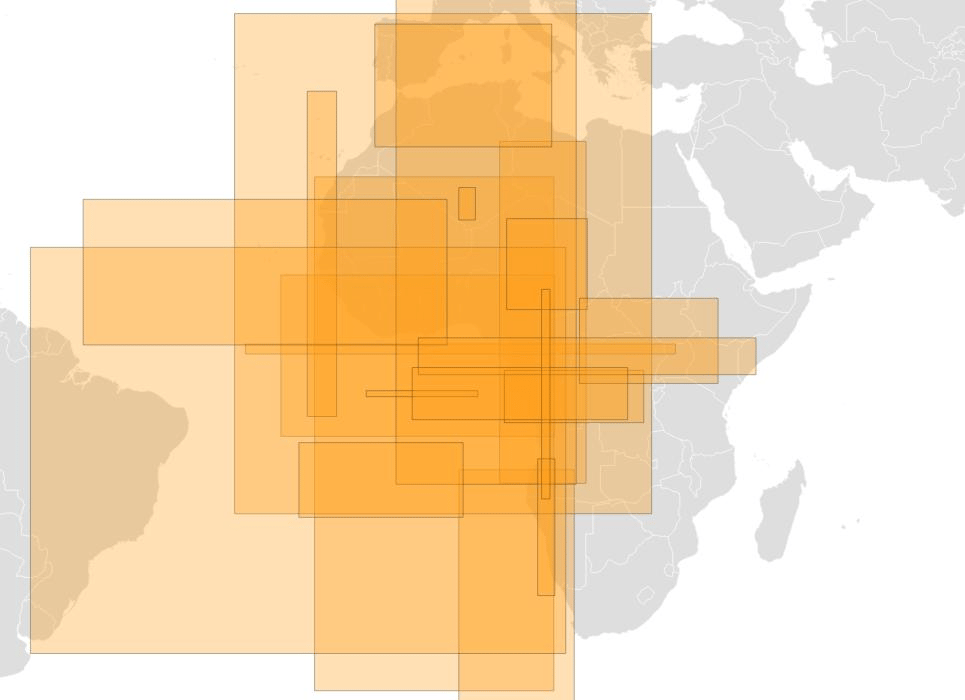But more interesting polygons have curvy and convex shapes, how can we generate those?

### Random Polygons with Concave Hull

One way is to extract a polygon from a set of random points, using ST_ConcaveHull(), and then applying an "erode and dilate" effect to make the curves more pleasantly round.

We start with a random center point for each polygon, and create a circle with ST_Buffer().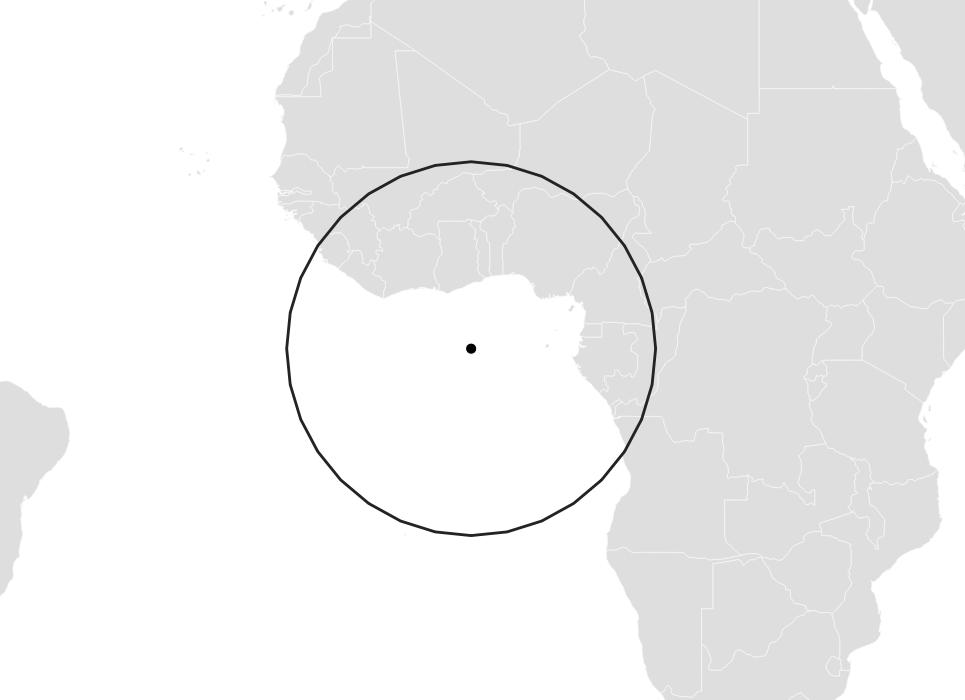Then use ST_GeneratePoints() to fill the circle with some random points -- not too many, so we get a nice jagged result.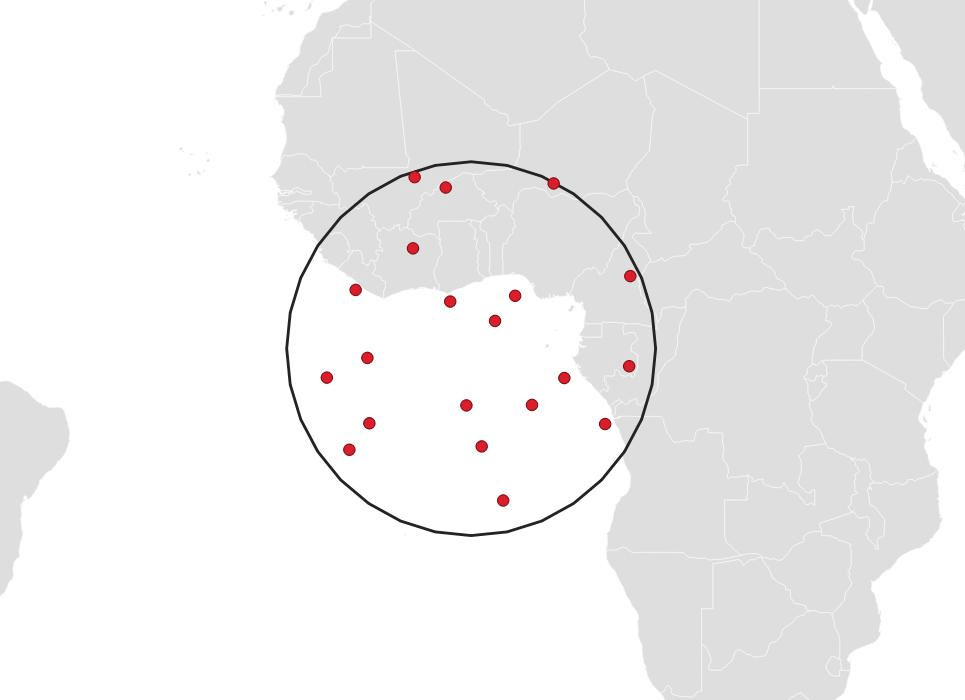Then use ST_ConcaveHull() to trace a "boundary" around those points.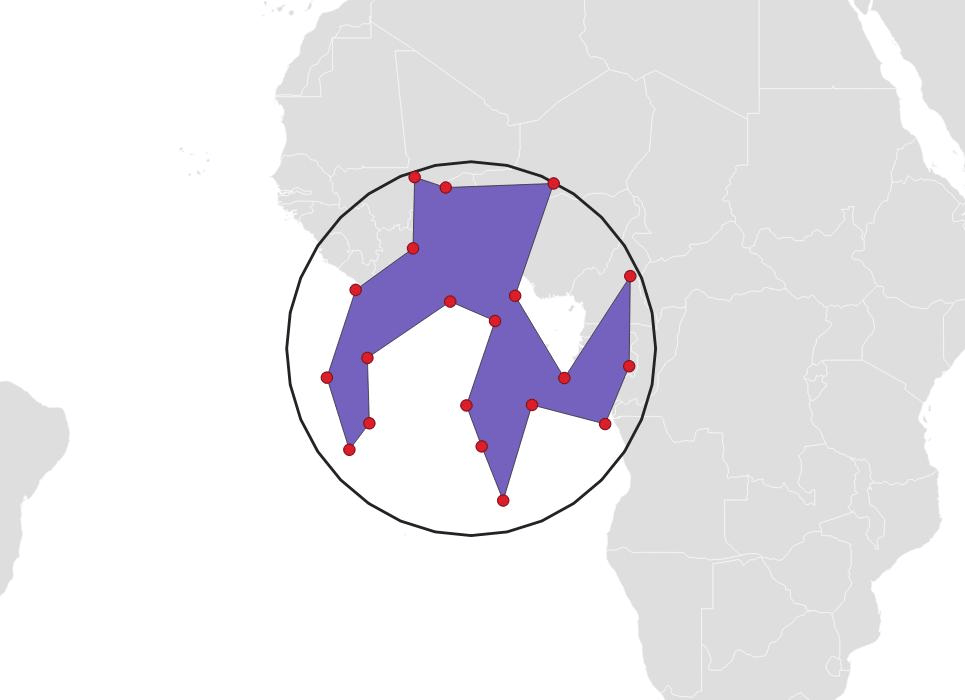Then apply a negative buffer, to erode the shape.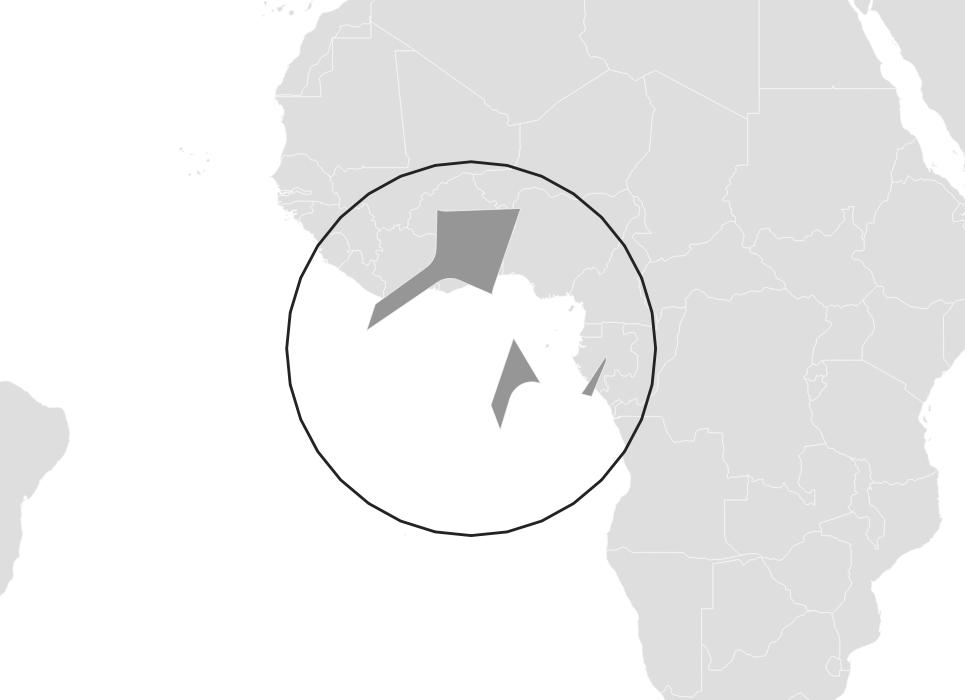And finally a positive buffer to dilate it back out again.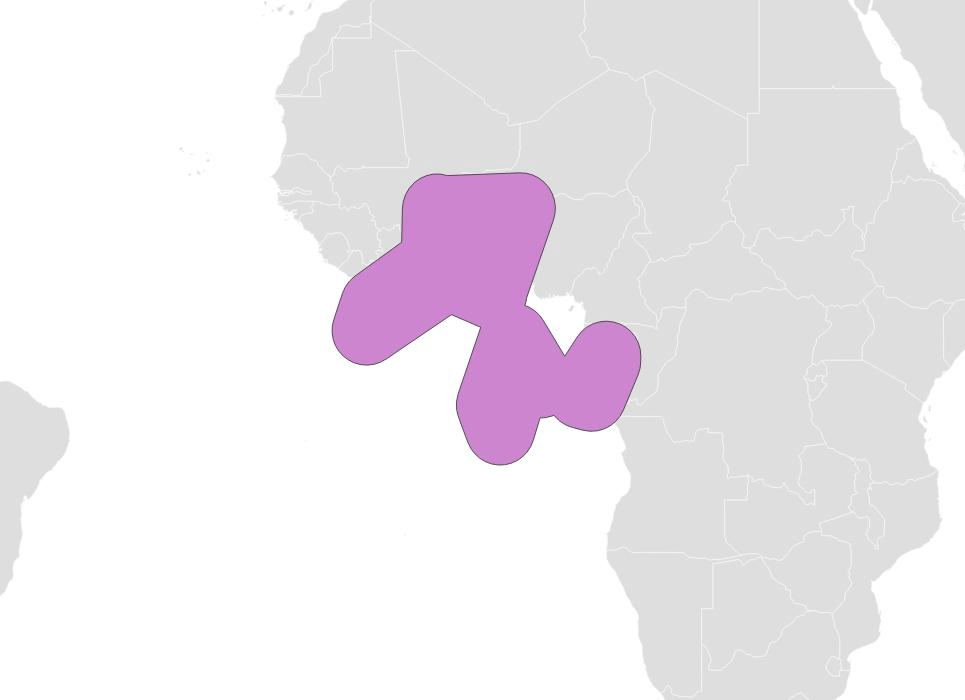Generating multiple hulls involves stringing together all the above operations with CTEs or subqueries.

Here is the full query to generate multiple polygons with the concave hull method.
``````CREATE TABLE random_hulls AS
WITH bounds AS (
SELECT 0 AS origin_x,
0 AS origin_y,
80 AS width,
80 AS height
),
polypts AS (
SELECT ST_Point(random_normal(origin_x, width/2),
random_normal(origin_y, width/2),
4326)::Geometry(Point, 4326) AS geom,
polyid
FROM bounds,
generate_series(1,10) AS polyid
),
pts AS (
SELECT ST_GeneratePoints(ST_Buffer(geom, width/5), 20) AS geom,
polyid
FROM bounds,
polypts
)
SELECT ST_Multi(ST_Buffer(
ST_Buffer(
ST_ConcaveHull(geom, 0.3),
-2.0),
3.0))::Geometry(MultiPolygon, 4326) AS geom,
polyid
FROM pts;
``````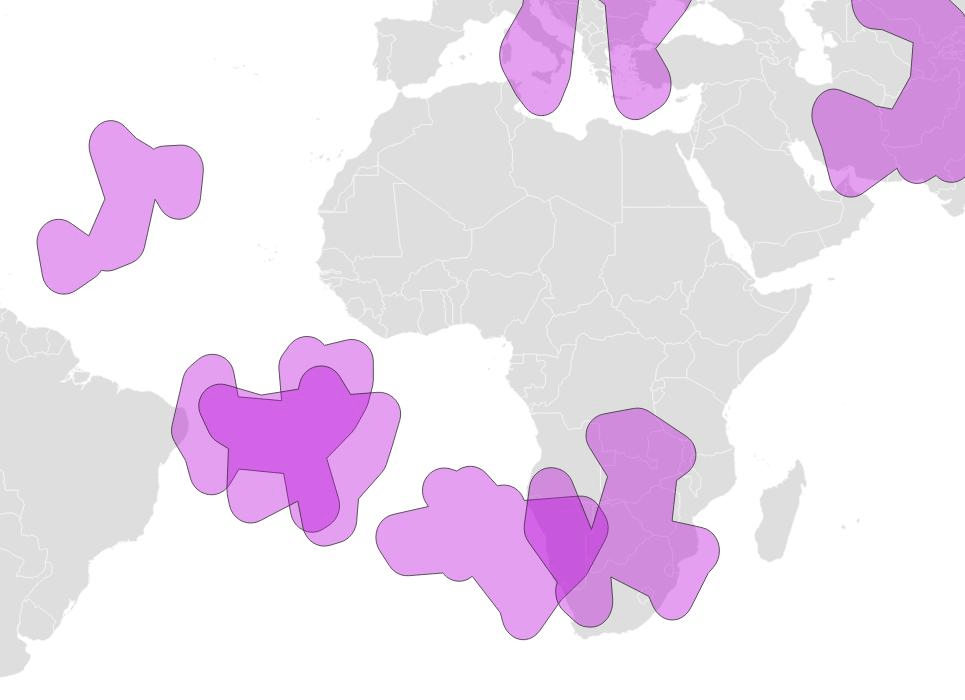### Random Polygons with Voronoi Polygons

Another approach is to again start with random points, but use the Voronoi diagram as the basis of the polygon.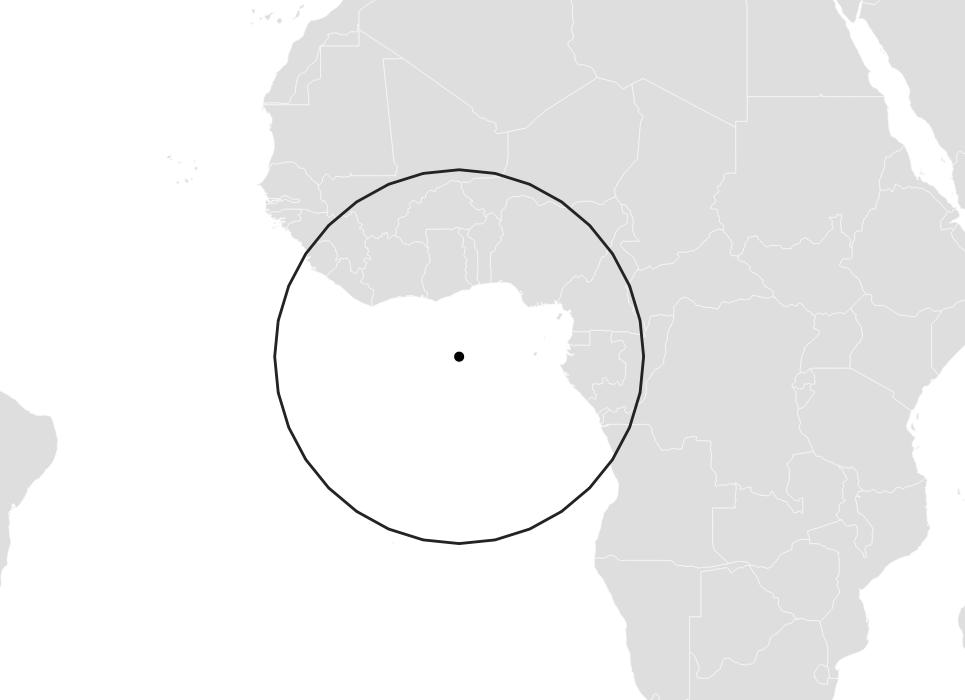Generate random points in the circle.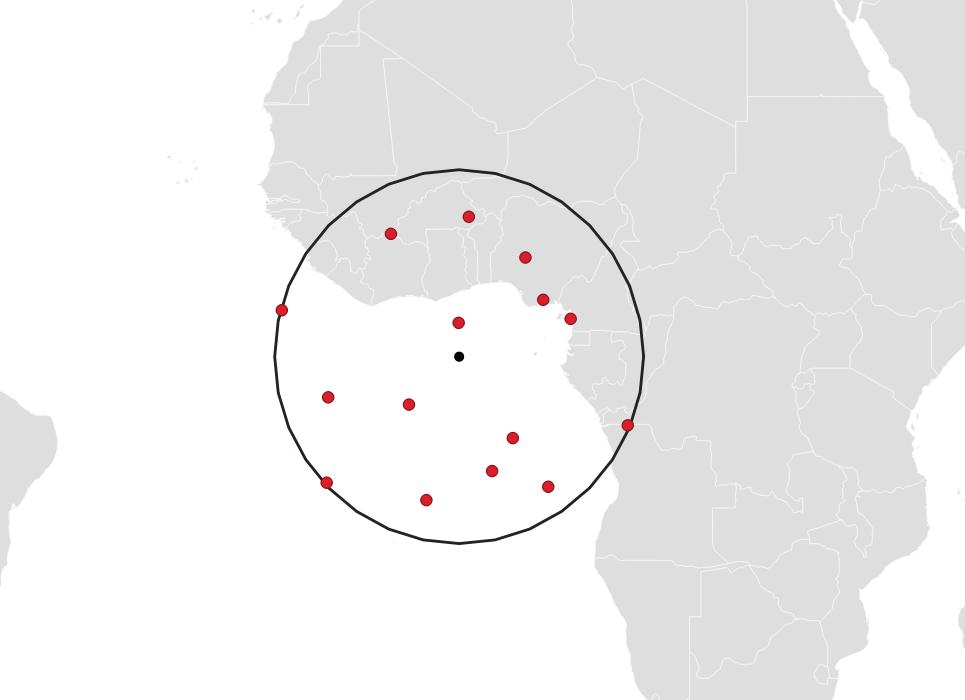Use the ST_VoronoiPolygons() function to generate polygons that subdivide the space using the random points as seeds.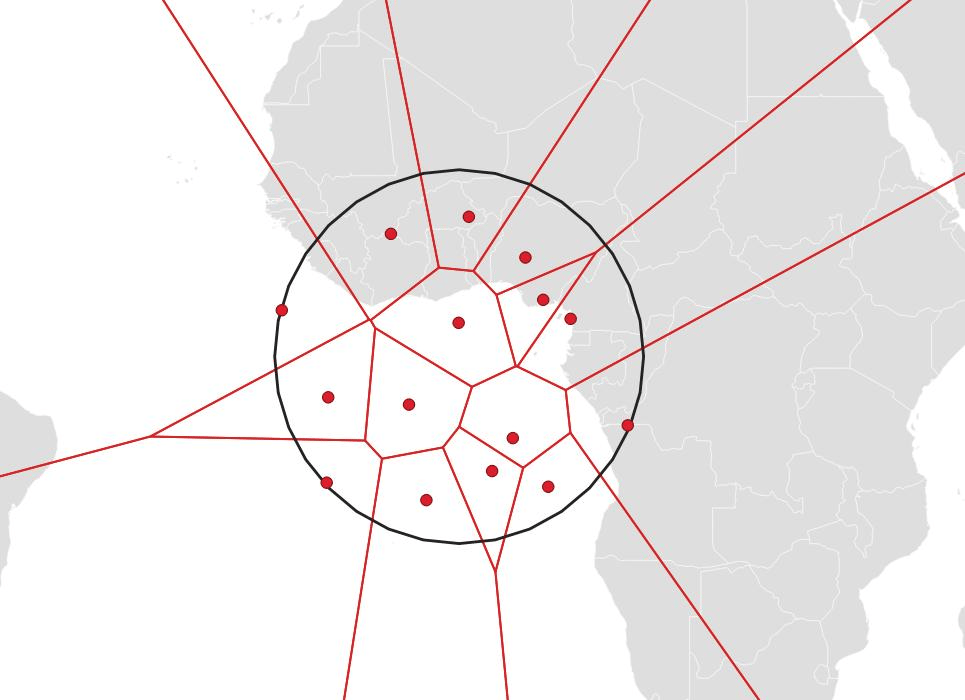Filter just the polygons that are fully contained in the originating circle.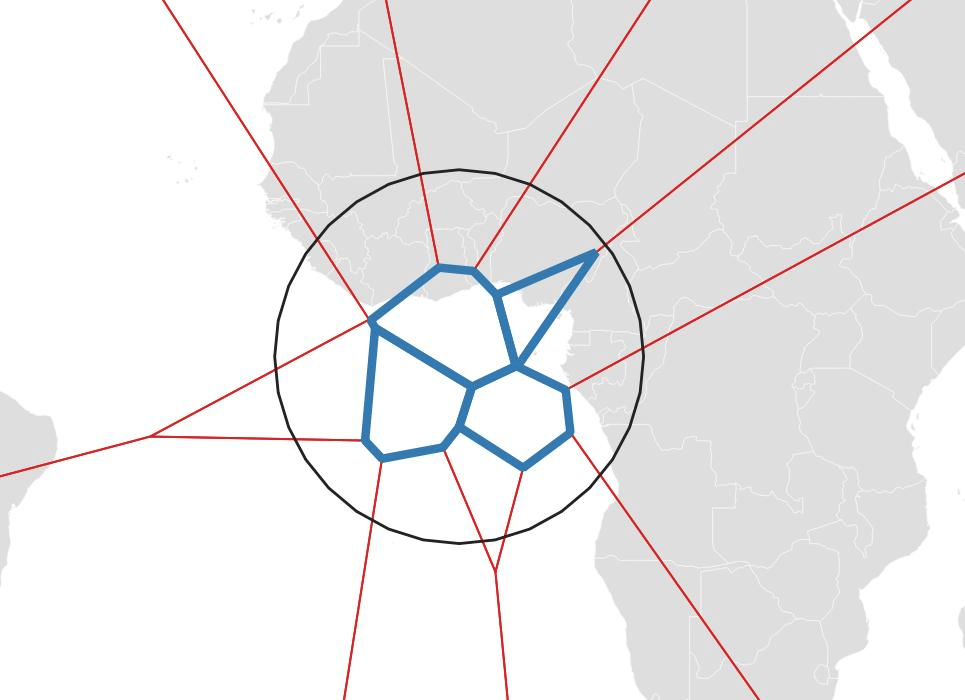And then use ST_Union() to merge those polygons into a single output shape.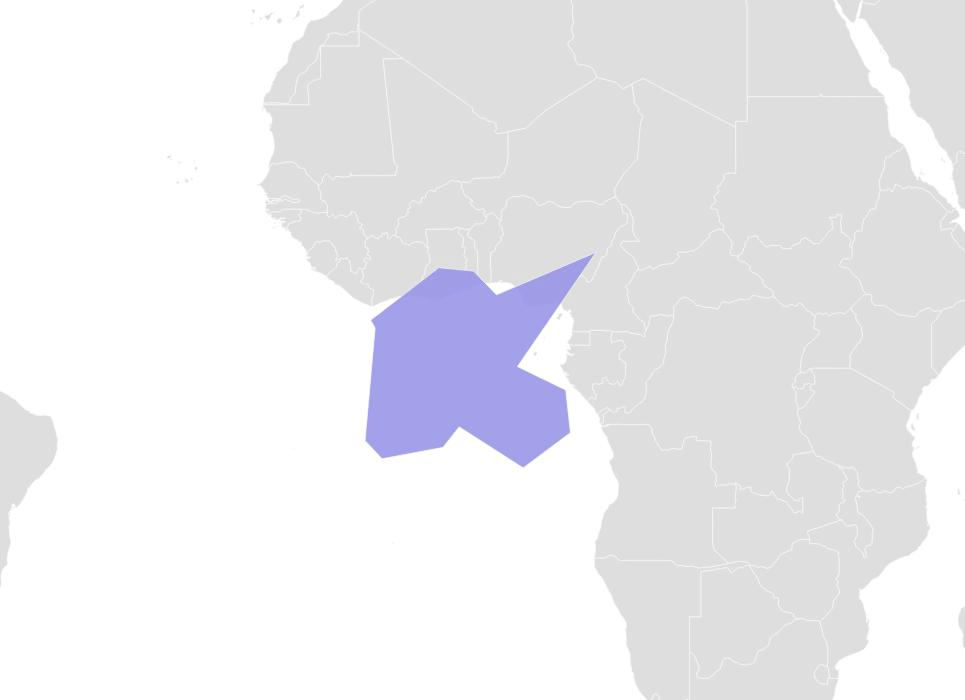Generating multiple hulls again involves stringing together the above operations with CTEs or subqueries.

Here is the full query to generate multiple polygons with the Voronoi method.
``````CREATE TABLE random_delaunay_hulls AS
WITH bounds AS (
SELECT 0 AS origin_x,
0 AS origin_y,
80 AS width,
80 AS height
),
polypts AS (
SELECT ST_Point(random_normal(origin_x, width/2),
random_normal(origin_y, width/2),
4326)::Geometry(Point, 4326) AS geom,
polyid
FROM bounds,
generate_series(1,20) AS polyid
),
vonorois AS (
SELECT ST_VoronoiPolygons(
ST_GeneratePoints(ST_Buffer(geom, width/5), 10)
) AS geom,
ST_Buffer(geom, width/5) AS geom_clip,
polyid
FROM bounds,
polypts
),
cells AS (
SELECT (ST_Dump(geom)).geom, polyid, geom_clip
FROM vonorois
)
SELECT ST_Union(geom)::Geometry(Polygon, 4326) AS geom, polyid
FROM cells
WHERE ST_Contains(geom_clip, geom)
GROUP BY polyid;
``````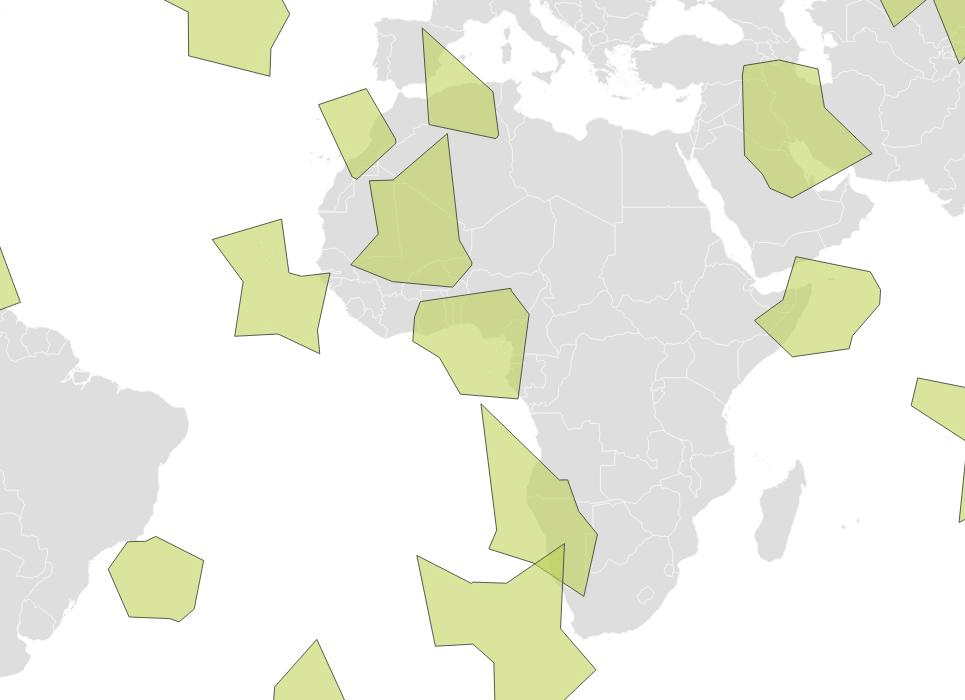Written by

Paul Ramsey

September 11, 2023 More by this author